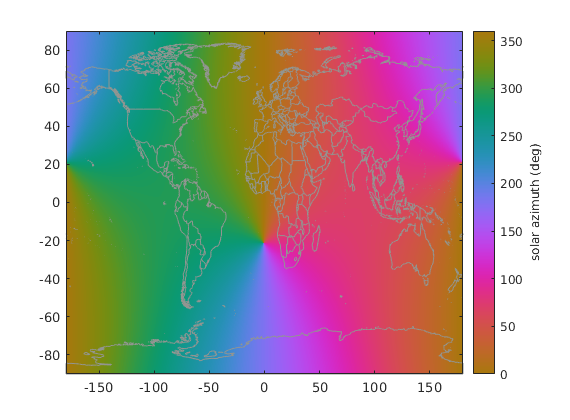# sun_angle documentation

sun_angle gives the solar azimuth and elevation for any time at any location on Earth. This function was adapted from the SolarAzEl function by Darin C. Koblick.

Back to Climate Data Tools Contents.

## Syntax

```[az,el] = sun_angle(t,lat,lon)
[az,el] = sun_angle(t,lat,lon,h)```

## Description

[az,el] = sun_angle(t,lat,lon) gives the solar azimuth and elevation in degrees at the specified geographic locations and times t in UTC. Input t can be datenum, datestr, or datetime format.

[az,el] = sun_angle(t,lat,lon,h) specifies height above sea level in meters. If no height is specified, elevations are automatically determined via the CDT function topo_interp.

## Example 1: Time series at one location

As I write this, I'm sitting at a coffee shop in Pasadena, California, (34.16N,118.13W). Determine the sun's azimuth and elevation right now and for the next 10 days.

```t = linspace(now,now+10,10000); % a time array 10 days long
t = t + 8/24; % converts Pacific Standard Time to UTC

[az,el] = sun_angle(t,34.16,-118.13);
```

Use subsubplot to plot the sun's angles:

```subsubplot(2,1,1)
plot(t,az)
axis tight
ylabel 'azimuth (deg)'
datetick('x','keeplimits')

subsubplot(2,1,2)
plot(t,el)
axis tight
ylabel 'elevation (deg)'
datetick('x','keeplimits')
```## Example 2: Global grid

Use cdtgrid to create a quarter-degree global grid and get the sun angles everywhere around the globe at the UTC strike of midnight on May 27, 2019:

```[lat,lon] = cdtgrid(1/4);

[az,el] = sun_angle('may 27, 1984 00:00:00',lat,lon);
```

Use imagescn to plot the solar azimuth and set the colormap to cmocean phase. Add political boundaries for context using borders.

```figure
imagescn(lon,lat,az)
cb = colorbar;
ylabel(cb,'solar azimuth (deg)')
caxis([0 360])
cmocean phase
borders('countries','color',rgb('gray'))
```Overlay sun elevation as contours. Of course, the sun elevation is only physical where the sun is above 0 elevation, so only include positive contours:

```hold on
contour(lon,lat,el,[0 0],'k') % solid zero contour
contour(lon,lat,el,0:10:90,'k:')

text(0,0,{'It''s night time';'everywhere south';'of the solid contour'},...
'horiz','center')
```## Example 3: The tiny effects of elevation

The sun's angle is affected by elevation above sea level. By default, sun_angle uses topo_interp to determine ground surface elevation above sea level if no elevation is specified. But here we consider a hypothetical tower, 10,000 meters tall in the center of London (51.51N, 0.123W). What's the sun azimuth and elevation along this tower at 7:45 pm on July 14, 2019?

```t = 'july 14, 2019 7:45 pm';
lat = 51.51;
lon = -0.123;
z = 0:10000; % height along tower (m)

[az,el] = sun_angle(t,lat,lon,z);

figure
subplot(1,2,1)
plot(az-az(1),z) % plot relative to base
axis tight
xlabel '\Delta azimuth (deg)'
ylabel 'elevation (m)'

subplot(1,2,2)
plot(el-el(1),z) % plot relative to base
axis tight
xlabel '\Delta elevation (deg)'
```Note the scientific notation on the x axis scales. That tells us the effect of elevation is miniscule on the scale of a few thousand meters.

## Author Info

This function was originally written by Darin C. Koblick using the formulas described here: http://stjarnhimlen.se/comp/tutorial.html#5. It was adapted for the Climate Data Toolbox for Matlab in 2019 by Chad A. Greene.